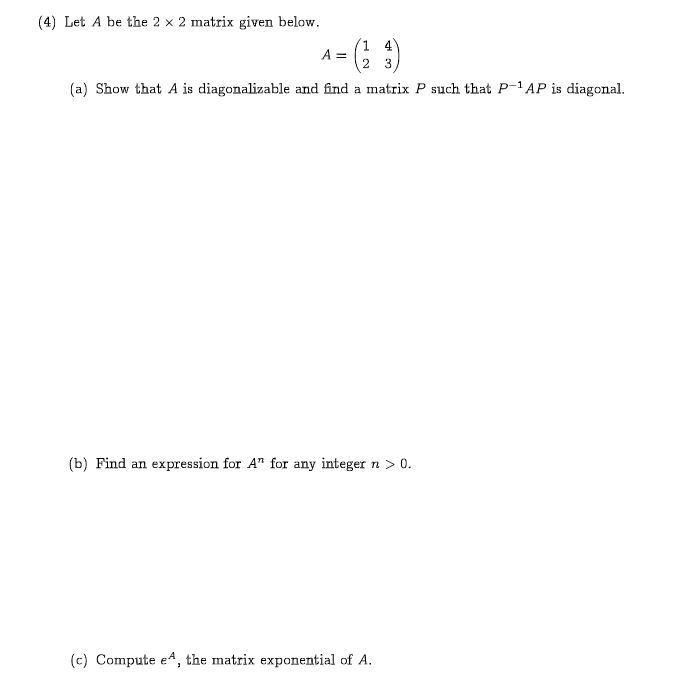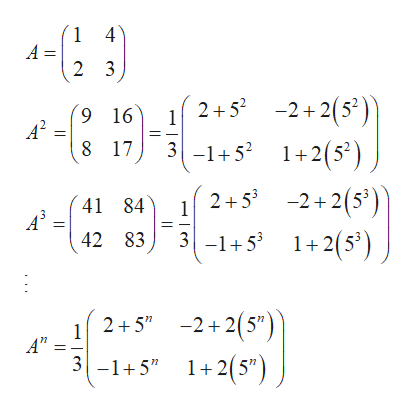# (4 Let A be the 2 x 2 matrix given below1 4A =23(a) Show that A is diagonalizable and find a matrix P such that P-1AP is diagonal.(b) Find an expression for A" for any integer n > 0.(c) Compute eA, the matrix exponential of A.

Question
1 viewshelp_outlineImage Transcriptionclose(4 Let A be the 2 x 2 matrix given below 1 4 A = 2 3 (a) Show that A is diagonalizable and find a matrix P such that P-1AP is diagonal. (b) Find an expression for A" for any integer n > 0. (c) Compute eA, the matrix exponential of A. fullscreen
check_circle

Step 1

Observe the general te...help_outlineImage Transcriptionclose1 4 A = 2 3 -2+2(52) 2+5 9 16 A2 1 8 17 3-1+5 1+2(5) 2+52+2(5 3 -1+51+2(5) 41 84 A3 1 42 83 -2+2(5" 1+2(5") 2+5" 1 A'" 3-1+5" fullscreen

### Want to see the full answer?

See Solution

#### Want to see this answer and more?

Solutions are written by subject experts who are available 24/7. Questions are typically answered within 1 hour.*

See Solution
*Response times may vary by subject and question.
Tagged in

### Math